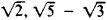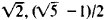algebraic number

(redirected from Algebraic numbers)
Also found in: Dictionary, Thesaurus.
Related to Algebraic numbers: Transcendental numbers

algebraic number:

see numbernumber,
entity describing the magnitude or position of a mathematical object or extensions of these concepts. The Natural Numbers

Cardinal numbers describe the size of a collection of objects; two such collections have the same (cardinal) number of objects if their
.

Algebraic Number

any number ɑ satisfying the algebraic equation a1 an + . . . + aka a + an+1 = 0, where n ≥ 1 and a1, . . . , an and an+1 are whole (rational) numbers. The number ɑ is called an algebraic integer if a1 = 1. If the polynomial f(x) = a1xn+ . . . + anx + an+1 is not the product of two other polynomials of positive degree with rational coefficients, then the number n is called the degree of the algebraic number a. The simplest algebraic numbers are the roots of the binomial equation xn = a, where α is a rational number. For example, algebraic numbers are the rational numbers and the numbersintegral algebraic numbers are the integers and the numbers. Two important trends in number theory are closely associated with the concept of the algebraic number. The first is the arithmetic of algebraic numbers (algebraic theory of numbers), originated by E. Kummer in the middle of the 19th century, which studies properties of algebraic numbers. Algebraic integers possess a number of properties analogous to those of integral rational numbers; however, the unique factorization theorem has no place in the theory of algebraic integers. To preserve unique factorization, Kummer introduced his so-called ideal numbers. The second trend is the theory of approximation of algebraic numbers, which studies the degree of approximation of these numbers by rational numbers or by algebraic numbers themselves. The first result in this direction was the theorem of J. Liouville, which showed that algebraic numbers are “badly” approximated by rational numbers. More precisely, if α is an algebraic number of degree n, then, for any integral rational values of ρ and q, the inequality ǀa − p/q ǀ > C/qn, holds, where C = C(a) > 0 is a constant independent of ρ and q, from which it follows that it is not difficult to construct an arbitrary number of nonalgebraic numbers—the transcendental numbers.

REFERENCES

Gekke, E. Lektsii po teorii algebraicheskikh chisel. Moscow-Leningrad, 1940. (Translated from German.)
Gel’fond, A. O. Transtsendentnye i algebraicheskie chista. Moscow, 1952.
Borevich, Z. I., and I. R. Shafarevich. Teoriia chisel. Moscow, 1964.

A. A. KARATSUBA

algebraic number

[¦al·jə¦brā·ik ′nəm·bər]
(mathematics)
Any root of a polynomial with rational coefficients.
References in periodicals archive ?
2] for the description of all algebraic numbers expressible as differences or as quotients of two conjugate algebraic numbers and ,  for some further work on this problem.
As already mentioned, it is proved in [BFK12] that any algebraic number is computable for k = 2 and q > 2 colors, but with the significant difference that the 2 balls may be turned into two different colors.
An algebraic number is one that can serve as a solution to a polynomial equation made up of x and powers of x.
Algebraic Number Theory and Fermat's Last Theorem, 4th Edition
Ono, An introduction to algebraic number theory, translated by the author from the second Japanese edition of Suron Josetsu, Plenum Press, New York, 1990.
In his text he covers algebraic numbers, field extensions, minimal polynomials, multiply generated fields, and the Galois correspondence, closing with such classical topics as binomial equations and solvability in radicals.

Site: Follow: Share:
Open / Close